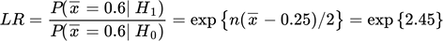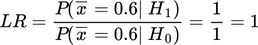## Introduction

###### The Likelihood Principle

The likelihood principle (LP) is principle of statistical inference. It was stated and proven in 1962 by Alan Birnbaum, and in the years since it has been ‘disproven’ and ‘affirmed’ multiple times. The ongoing battle over whether the LP follows from two fundamental frequentist principles is a technical battle that, in my opinion, has overshadowed its broader implication.

The essence of the LP is this: same data, same evidence. A less technical but more precise version is: If two experiments yield data such that their likelihood functions are proportional, then those two sets of data represent equivalent instances of statistical evidence.

The likelihood function is the most compact summary of the data that does not loose information. When two likelihood functions are equal, or proportional to each other, their information content is identical. This means, for example, that both data sets will yield the same parameter and variance estimates. It seems natural then, that the assessment of the statistical evidence, however it is accomplished, should also be identical in the two data sets.

What the LP does not say is worth noting. It does not say that the two studies yielding proportional likelihoods must have had the same error rates. It does not say that the likelihood function should be used to measure the strength of statistical evidence. In fact, the LP does not even specify how to measure of the strength of statistical evidence. It says only that the measurement of the strength of evidence, whatever it is, should yield the same answer when the likelihood functions are proportional.

The LP is often mistaken for the Law of Likelihood (LL), which is an axiom advancing the use of likelihood ratios as measures of evidence (Royall 1997, Hacking 1965). LP and LL are often conflated, as if there was only one guiding principle. However, the LP applies to all measures of statistical evidence: when the likelihoods are proportional, the statistical evidence is equivalent.

## Overview

###### The General Case

An important corollary of the LP is that two instances of equivalent statistical evidence will have the same propensity to be misleading – i.e., their false discovery rates (FDR) will be equal (when the prior is fixed). The reliance of the FDR on likelihood function is important.

Let x represent a summary of the data or the data themselves. We will consider two hypotheses, H1 and H0, where r=P(H1)/P(H0). There is no loss of generalizability in using only two simple hypotheses and its simpler to do the math. The likelihood ratio isHere P(x|H) is the probability of observing the data we actually collected, x, assuming hypothesis H is true. The likelihood ratio is comparing the ability of hypotheses to predict what actually happened.

Now, upon observing data x and finding that it supports the alternative over the null, i.e. that LR>1, we would like to know the chance that the data are mistaken. This propensity is given by the false discovery rateWhen x supports the null over the alternative, i.e. when LR<1, we would be interested in the false confirmation rate FCR=P(H1|x) instead, but it is sufficient for our purposes to focus on the FDR here. Notice that the false discovery rate is driven to zero as the evidence accumulates in favor of the alternative hypothesis, i.e. as the LR grows large.

## Examples

###### 5 Scenarios

Let’s consider an example to see how this all plays out. Suppose we collect 14 observations from a normal distribution where the standard deviation is known to be one. The null hypothesis is that the population mean is 0 and the alternative hypothesis is that the population mean is ½. We do not a priori favor either hypothesis, so r=1. The plan is to collect data and reject the null hypothesis if the sample mean is greater than ½. The Type I error rate is 0.031 and the power is 0.5.

Scenario 1: Upon collecting data, we reject the null hypothesis. In this instance, nothing but the result of the hypothesis test is communicated, so the data summary we have is x={Reject H0}. The likelihood ratio based on this summary iswhich is 16.13 (=0.5/0.031). It follows that the false discovery rate (FDR) is 1 / (1 + 16.13) =0.058. The study had a 3.1% chance rejecting a true null hypothesis and we rejected it. The classical reasoning here is that either we have made a mistake or the alternative hypothesis is correct. The chance that the observed rejection is a mistake is given by the FDR at 5.8%.

Scenario 2: Things change slightly if the p-value, say 0.0124, is reported because it conveys additional information than just the result of the hypothesis test. Now the data summary is x={p=0.0124} and the likelihood ratio isHere (1-beta_alpha=0.0124) is the power of the test when the Type I Error rate is set to 0.0124. This is readily computed, and we find that LR =28.78 =0.354/0.0123, which is larger than before. As a result, the false discovery rate decreases to 0.034 =1 / (1 + 28.78).

Scenario 3: The p-value can change even when your data does not. For example, had we also performed three other tests at the same time, a multiple comparisons correction would be required. The Bonferroni corrected p-value is 0.0372 = min(3*p, 1). Now the likelihood ratio changes towhich is only 14.4 (=0.534/0.037) and comes with an increased FDR of 0.065 =1 / (1 + 14.4). Of course, a different correction yields a different p-value and a different FDR. The awkwardness is not that the FDR changes with the p-value, but that these changes happen while the data remain constant.

Scenario 4: Now suppose that an observed sample mean of 0.6 is reported. Its (unadjusted) p-value is 0.0124, which is scenario 2. But the sample mean is a minimal sufficient statistic, meaning that it is the most compact summary of the data that does not loose information (Recall that we know the variance. If we did not, the MSS would be the sample mean and sample variance). Now the data is x={sample mean of 0.6} and the likelihood ratio iswhich is 11.588 when the observed sample mean is 0.6 (Blume, 2002, eqn. 8). It follows that the false discovery rate is 8% because 1 / (1 + 11.588) = 0.079.

The graphs below show what is happening. The graph on the left shows how the likelihood ratio for H1 versus H0 from each scenario changes with the alternative hypothesis. Likewise, I’ve plotted the corresponding false discovery rate (when LR>1) to show how that changes with the alternative.We can see from these graphs how the p-value summary distorts the assessment of evidence. The red curve implies that all population means greater than 1.5 (e.g. H2: population mean = 1 Billion) are better supported by the data over the null hypothesis. This is clearly silly. With an observed sample mean of only 0.6, population means of 1 Billion are bit of a stretch, to say the least. Nevertheless, the red false discovery rate curve implies it is a near certainty. This distortion is to be expected given the nature of the p-value. The proper representation of evidence and FDR is given by the blue curve.

Scenario 5: Cheaters never win unless they also lie. Suppose the entire experiment is repeated until a sample mean of 0.60 (rounded to two digits) is observed and then the sample mean of 0.6 is presented as a summary of the data. This would entail repeatedly collecting groups of 14 observations and throwing them away until we find one where sample mean is 0.6. What is the likelihood ratio and false discovery rate?

A common mistake is to assume that the analysis in scenario 4 would apply and yield a likelihood ratio of 11.588 and FDR of 8%. In scenario 4, the data were drawn at random from a normal distribution; but this is no longer the case. Consequently, the likelihood calculations from scenario 4 are no longer correct. The correct likelihood ratio is 1:Why? Because, the sampling plan was to repeat the experiment until sample mean of 0.60 was observed. So, it must be that P(sample mean=0.6|H1)=1 and P(sample mean=0.6|H0)=1. Consequently, the false discovery rate is just P(H0) because FDR=1 / (1+r). This indicates that the data are utterly worthless because of how they were obtained.

The only way the likelihood assessment of the statistical evidence goes astray is if the sampling plan is never revealed and the wrong likelihood function is used. But this is precisely when every other assessment, Frequentist and Bayesian, also goes astray. Cheaters never win unless they lie.

Adjusting likelihoods for sampling schemes is not unusual in statistics, but it is an often overlooked point. Also, an urge to adjust a p-value does not always mean the sampling scheme is problematic. Take, for example, a study with 4 endpoints. If the data collection is effectively independent across the endpoints, then it is fine to use the same likelihood that one would have used if that endpoint was the only one under consideration.

The point is that two studies that yield identical data sets don’t necessarily yield identical likelihood function. And when likelihood changes, the FDRs differ.